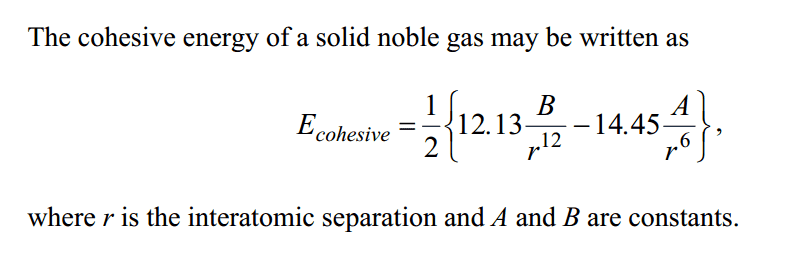# Lennard-Jones Potential: Derive an expression for equilibrium constant

Flucky
Edit: well this is frustrating, not sure why the itex things aren't working..

Edit2: I've attached a picture of the question at the bottom as well.

## Homework Statement

The cohesive energy of a solid noble gas may be written as

(See picture below)

Derive an expression for the equilibrium separation r0 of the atoms.

## The Attempt at a Solution

At Ecohesive, r=r0 and dE/dr0 = 0

So first I rearranged the main equation into something nicer to differentiate:

E = 6.065Br$^{-12}_{0}$ - 7.225Ar$^{-6}_{0}$

Then differentiated:

dE/dr0 = -72.78$\frac{B}{r^{13}_{0}}$ + 43.35$\frac{A}{r^{7}_{0}}$ = 0

43.35$\frac{A}{r^{7}_{0}}$ = 72.78$\frac{B}{r^{13}_{0}}$

r$^{6}_{0}$ = $\frac{72.78}{43.35}$$\frac{B}{A}$

So r0 = ($\frac{72.78}{43.35}$$\frac{B}{A}$)$\frac{1}{6}$

But this looks pretty horrible, is it any good?

Cheers.

-------------------------------------------------------------------------------------------Last edited: